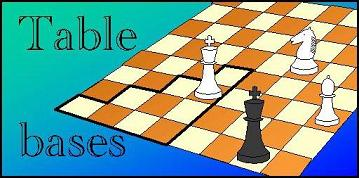Leapfrog: Packing Bitmaps# Compressing Sparse Bitmaps

### Run-Length Coding

We use a rather simple compression algorithm, that stores the packed data as a sequence of bytes. Each byte codes for zero, one or two lost positions, as follows: Codes 0-189 encode the next two lost positions, when their two run lengths totals 18 or less. For example, code 0 means 0+0 run length (so two immediate consecutive ones), code 1 means 0+1 (101...), code 2 1+0 (011...) etc., until code 189 means 18+0 (00000000000000000011...). codes 191-255 are used to encode a single lost position, where code 191 means it immediately follows (run length 0), code 192 means it has run-length 1, and so on until code 255 means run length 64. Note that single-position codes with a run-length of 18 or smaller imply a certain number of zero bits after the lost position they code for as well, or a double code could have been used. So code 193 means run length 2, but as there are double codes for 2+n upto n=16, the latter meaning 001[16 zeros]1, the fact that none of these was used implies 17 zeros must follow, i.e. code 193 means 001[17 zeros]. Such trailing zeros then do not have to be encoded in the next symbol, enhancing the chances that a more compact encoding can be used. Finally, code 190 means 64 zeros, and is used as a spacer when the next lost position is out of range of even the single encodings.

If this coding scheme is used on totally random scatterings of one-bits, making up a fraction f of the total (the rest being zero bits), the probability that a run length cannot be encoded in a single byte is (1-f)^64.

To make an estimate of what compression to expect, and hence how much array space to reserve for the packed lists, we note that Lost positions are not expected to total more than about 50% of all positons. A single piece of black already covers some 8-20% of the board, and if a white King hapens to be on that 10%, black to move is certainly not lost. And black is supposed to have 3 or 4 pieces in the tablebases we are building. Furthermore, if another white piece is under fire, it is likely to be undefended, and capturing it usually also spoils the win for white. And white is also supposed to have 2-4 pieces. So 30-50% lost positions is actually very high, even for a totally won end-game.

### Compression Factor

As each 4-men disk chunk has 16M positions, encoding the run-length between the positions by 1 bytes, if half of them are won, would require 8MB. Encoding them by 2 bytes would require 16MB. But as a single byte works upto run length of 190, we can only need 2 bytes for every position in a lost(N) set if only 0.5% of the positions are lost(N), which would require then 100 cycles to make every position lost. Usually the bulk of the lost positions is concentrated in less than 10 cycles, and these cycles then have 5% of the positions declared as lost(N), implying an everage distance between them of only 20, so that 1 byte is likely to be enough to encode the bulk of the lost positions. We reserve 13MB on the disk per 4-men chunk, which should be enough to hold all packed lists for all lost(N). With the 2MB Won bitmap, we then need 15MB disk storage per chunk. For a 7-men pawnless tablebase we have 1/8 * 64^3 = 32K 4-men chunks, for a total size of 480 GB. This fits nicely on a 500GB hard drive, so there is no reason to economize further.

The other concern is the memory requirement of a single lost(N) list. Such a list is biggest when a very large fraction of the positions is in a single lost(N) set. As remarked, hardly ever more than 10% of all positions is lost(N) for any single N. In such a case the average spacing between lost(N) positions is only 10 positions, and virtually all run length will have single-byte encodings. With 10% of the positions requiring 8 bits, we have 0.8 bit per position, still making the lost(N) packed list smaller than the bitmap it represents (which uses 1 bit per position), even with this primitive encoding. But not by much. For most cycles it will be very much smaller, though.